# Color Image Denoising with Median Filtering¶

Important: Please read the installation page for details about how to install the toolboxes. $\newcommand{\dotp}{\langle #1, #2 \rangle}$ $\newcommand{\enscond}{\lbrace #1, #2 \rbrace}$ $\newcommand{\pd}{ \frac{ \partial #1}{\partial #2} }$ $\newcommand{\umin}{\underset{#1}{\min}\;}$ $\newcommand{\umax}{\underset{#1}{\max}\;}$ $\newcommand{\umin}{\underset{#1}{\min}\;}$ $\newcommand{\uargmin}{\underset{#1}{argmin}\;}$ $\newcommand{\norm}{\|#1\|}$ $\newcommand{\abs}{\left|#1\right|}$ $\newcommand{\choice}{ \left\{ \begin{array}{l} #1 \end{array} \right. }$ $\newcommand{\pa}{\left(#1\right)}$ $\newcommand{\diag}{{diag}\left( #1 \right)}$ $\newcommand{\qandq}{\quad\text{and}\quad}$ $\newcommand{\qwhereq}{\quad\text{where}\quad}$ $\newcommand{\qifq}{ \quad \text{if} \quad }$ $\newcommand{\qarrq}{ \quad \Longrightarrow \quad }$ $\newcommand{\ZZ}{\mathbb{Z}}$ $\newcommand{\CC}{\mathbb{C}}$ $\newcommand{\RR}{\mathbb{R}}$ $\newcommand{\EE}{\mathbb{E}}$ $\newcommand{\Zz}{\mathcal{Z}}$ $\newcommand{\Ww}{\mathcal{W}}$ $\newcommand{\Vv}{\mathcal{V}}$ $\newcommand{\Nn}{\mathcal{N}}$ $\newcommand{\NN}{\mathcal{N}}$ $\newcommand{\Hh}{\mathcal{H}}$ $\newcommand{\Bb}{\mathcal{B}}$ $\newcommand{\Ee}{\mathcal{E}}$ $\newcommand{\Cc}{\mathcal{C}}$ $\newcommand{\Gg}{\mathcal{G}}$ $\newcommand{\Ss}{\mathcal{S}}$ $\newcommand{\Pp}{\mathcal{P}}$ $\newcommand{\Ff}{\mathcal{F}}$ $\newcommand{\Xx}{\mathcal{X}}$ $\newcommand{\Mm}{\mathcal{M}}$ $\newcommand{\Ii}{\mathcal{I}}$ $\newcommand{\Dd}{\mathcal{D}}$ $\newcommand{\Ll}{\mathcal{L}}$ $\newcommand{\Tt}{\mathcal{T}}$ $\newcommand{\si}{\sigma}$ $\newcommand{\al}{\alpha}$ $\newcommand{\la}{\lambda}$ $\newcommand{\ga}{\gamma}$ $\newcommand{\Ga}{\Gamma}$ $\newcommand{\La}{\Lambda}$ $\newcommand{\si}{\sigma}$ $\newcommand{\Si}{\Sigma}$ $\newcommand{\be}{\beta}$ $\newcommand{\de}{\delta}$ $\newcommand{\De}{\Delta}$ $\newcommand{\phi}{\varphi}$ $\newcommand{\th}{\theta}$ $\newcommand{\om}{\omega}$ $\newcommand{\Om}{\Omega}$

This numerical tour explores denoising of color images using a local multi-dimensional median. This is the sequel to the numerical tour <../tv_median/ Outliers and Median Denoiser>.

In :
addpath('toolbox_signal')


## Multidimensional Median¶

The median of |n| real values |x| is obtained by taking |v(n/2)| with |v=sort(x)| (with a special care for an even number |n|). It can alternatively obtained by minizing over |y| the sum of absolute values.

|\sum_i abs(x(i)-y)|

This should be contrasted with the mean that minimizes the sum of squares.

|\sum_i (x(i)-y)^2|

This allows one to define a mutidimensional median for set of points |x(i)| in dimension |d| by replacing |abs| by the d-dimensional norm.

We define a Gaussian point cloud in 2D.

In :
d = 2; % dimension
n = 1000; % number of points
X = randn(d,n);


We modify some points as positive outliers (to shift the mean).

In :
p = 100; % number of outliers
sel = randperm(n); sel = sel(1:p); % index of outliers
X(:,sel) = rand(d,p)*50;


We can compute the mean point.

In :
m = mean(X,2);


To compute the median in 2D, one needs to minimize the sum of norms. This is not as straightforward as the sum of squares, since there is no close form solution. One needs to use an iterative algorithm, for instance the re-weighted least squares, that computes weighted means.

number of iterations of the method

In :
niter = 30;


initialize the median using the mean

In :
med = m;
energy = [];
for i=1:niter
% comute the distance from med to the points
dist = sqrt( sum( (X-repmat(med,[1 n])).^2 ) );
% compute the weight, take care of not dividing by 0
weight = 1./max( dist, 1e-10 ); weight = weight/sum(weight);
% compute the weighted mean
med = sum( repmat(weight,[d 1]).*X, 2 );
energy(end+1) = sum( dist );
end


We can display the decay of the L1 energy through the iterations.

In :
clf;
plot(energy, '.-'); axis('tight')
set_label('Iteration', 'L1 energy');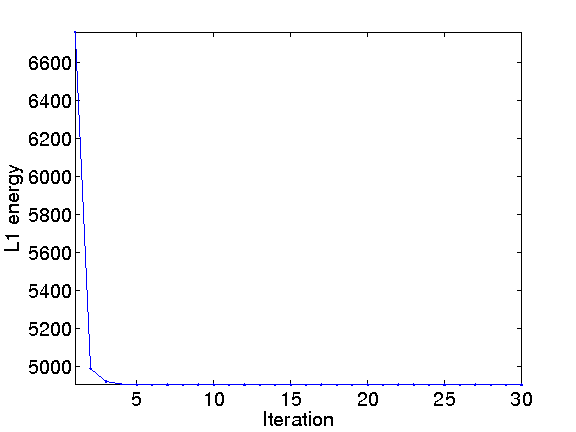We can display the points, the mean and the median.

In :
clf;
hold('on');
plot(X(1,:), X(2,:), '.');
plot(m(1,:), m(2,:), 'k*');
plot(med(1,:), med(2,:), 'ro');
axis('tight');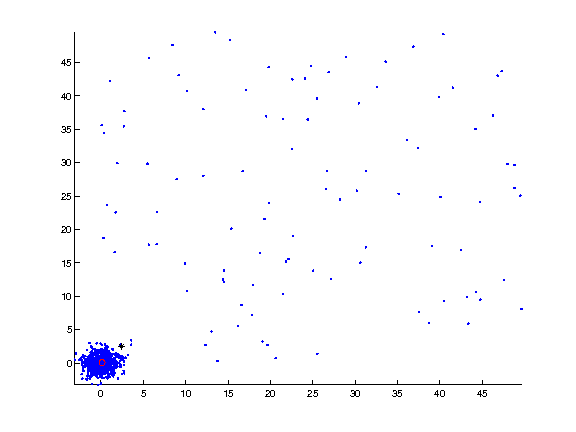## Color Image Denoising using 1D Median¶

A median filter can be used to denoise a color image, by applying it to each channel of the image.

We load a color image, which is an array of size |[n,n,3]|.

In :
name = 'flowers';
options.nbdims = 3;
n = 256;
M0 = rescale(M0);


We create a colored impulse noise by taking two Gaussians of different standard deviations.

percent of strong Gaussian

In :
rho = .4;


mask of pixel corrupted by strong gaussian

In :
mask = repmat(rand(n,n)<rho, [1 1 3]);


deviation of the two Gaussian

In :
sigma1 = .03; sigma2 = 1;


noise with two different Gaussians

In :
noise = sigma1*randn(n,n,3).*(1-mask) + sigma2*rand(n,n,3).*mask;


Add the noise to the image.

In :
M = M0+noise;
pnoisy = snr(M0,M);


Display the clean and noisy images.

In :
clf;
imageplot(M0, 'Clean image', 1,2,1);
imageplot(clamp(M), strcat(['Noisy, SNR=' num2str(pnoisy)]), 1,2,2 );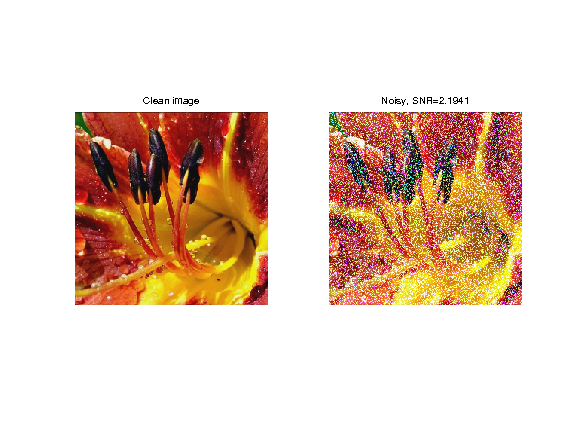In the following, we use a fixed window width.

In :
k = 4;
w = 2*k+1;


Exercise 1

A first way to denoise the image is to apply the local median filter implemented with the function |perform_median_filtering| on each channel |M(:,:,i)| of the image, to get a denoised image |Mindep| with SNR |pindep|.

In :
exo1()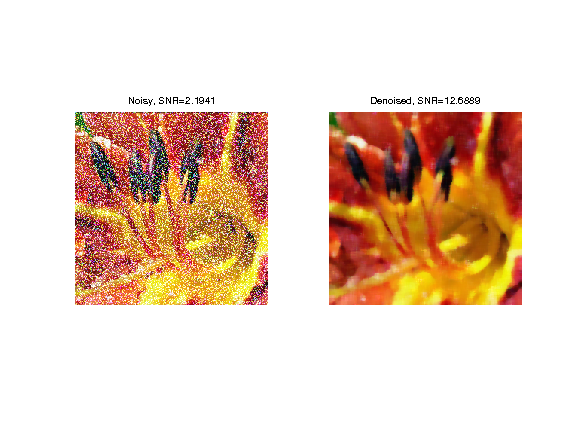In :
%% Insert your code here.


## Color Image Denoising using 3D Median¶

Another method computes a multidimensional median for patches located around each pixel of the image.

First we extract the 3D points corresponding to the colors in the patch located around a pixel at a location |(x,y)|.

example of pixel location

In :
x = 100; y = 73;


location of the patch, with pediodic boundary condition

In :
selx = x-k:x+k; selx = mod(selx-1,n)+1;
sely = y-k:y+k; sely = mod(sely-1,n)+1;


extract the patch

In :
patch = M(selx,sely,:);


patch of pixels, stored as a matrix

In :
X = reshape( patch, [w*w 3])';


Exercise 2

Compute the median |med| of the points in |X| using the iterative reweighted least squares algorithm. This computed median |med| should be stored in the result as |Mmed(x,y,:)| (you need to reshape |med| so that its size is |[1 1 3]|).

In :
exo2()

In :
%% Insert your code here.


We can display the points, the mean and the median, in 3D.

In :
m = mean(X, 2);
clf;
hold('on');
plot3(X(1,:), X(2,:), X(3,:), '.');
plot3(m(1), m(2), m(3), '*k');
plot3(med(1), med(2), med(3), 'or');
view(3); axis('tight');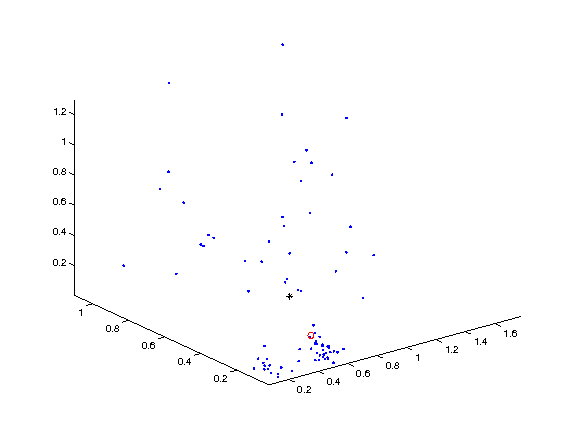Exercise 3

Implement the 3D median filter by looping through all the pixel |(x,y)|. isplay the results

In :
exo3()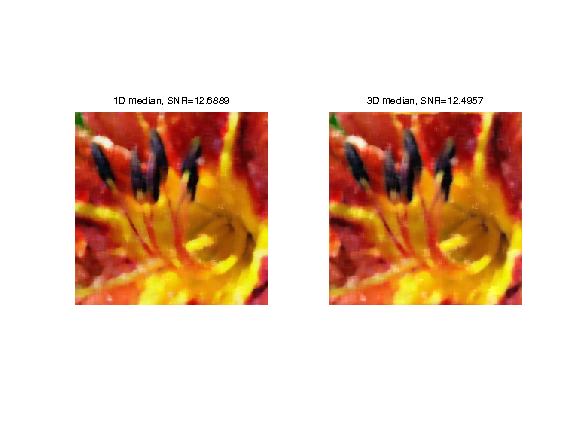In :
%% Insert your code here.